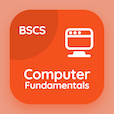Online Engineering Courses

Engineering Mathematics Practice Tests

Learn Online Engineering Mathematics Frequently Asked Questions

# Higher Level Mathematics Practice Problems with Solutions PDF

The e-Book Higher Level Mathematics Practice Problems with Solutions, Engineering Mathematics Multiple Choice Questions (MCQ Quiz) PDF download to learn online engineering mathematics courses. Solve Fourier Analysis Multiple Choice Questions and Answers (MCQs), Engineering Mathematics quiz answers PDF for job placement test. The eBook Higher Level Mathematics Practice Problems with Solutions App Download: differential equations final exam questions with solutions, advanced maths quiz questions with answers, differential equations questions with solutions, differential equations final exam with solutions test prep for questions to ask during an interview.

The MCQ: The computation and study of Fourier series is known as PDF, "Higher Level Mathematics Practice Problems with Solutions" App Download (Free) with linear analysis, harmonic analysis, homogeneous analysis, and symmetric analysis choices for job placement test. Practice higher level mathematics practice problems with solutions quiz questions, download Google eBook (Free Sample) for online colleges enrolling.

## Engineering Mathematics MCQs: Higher Level Mathematics Practice Problems with Solutions

MCQ: The computation and study of Fourier series is known as

A) Linear analysis
B) Harmonic analysis
C) Homogeneous analysis
D) Symmetric analysis

MCQ: An expansion of a periodic function f(x) in terms of an infinite sum of sines and cosines is called

A) Laplace transform
B) Fourier transform
C) integration
D) differentiation

MCQ: Series obtained from cosine and sine system is called

A) power series
B) laplacian series
C) trigonometric series
D) velocity series

MCQ: Cosine and sine functions have period of

A) π
B) 2π
C) π/2
D) π/4

MCQ: The sum of part of the sequence is called

A) differential sum
B) integral sum
C) partial sum
D) unit sum

### Practice Tests: Engineering Mathematics Exam Prep

Download Engineering Math Quiz App, Software Engineering MCQ App, and ICT MCQs App to install for Android & iOS devices. These Apps include complete analytics of real time attempts with interactive assessments. Download Play Store & App Store Apps & Enjoy 100% functionality with subscriptions!